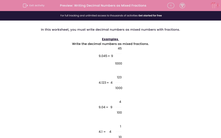# Writing Decimal Numbers as Mixed Fractions

In this worksheet, students write decimal numbers as mixed fractions.Key stage:  KS 2

Curriculum topic:   Maths and Numerical Reasoning

Curriculum subtopic:   Decimals

Difficulty level:#### Worksheet Overview

In this worksheet, you must write decimal numbers as mixed numbers with fractions.

Examples

Write the decimal numbers as mixed fractions.

9.045 = 9
45
1000

4.123 = 4
123
1000

9.04 = 9
4
100

4.1 = 4
1
10

### What is EdPlace?

We're your National Curriculum aligned online education content provider helping each child succeed in English, maths and science from year 1 to GCSE. With an EdPlace account you’ll be able to track and measure progress, helping each child achieve their best. We build confidence and attainment by personalising each child’s learning at a level that suits them.

Get started••••